Tension on the string at point P is T. The graph for tension (T) versus x is shown in the figure. Then the string is: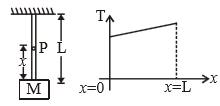1.  massless

2.  massfull

3.  tension on every point on the string is same when the string is having finite mass 4

4.  None of these

Concept Questions :-

Application of laws
High Yielding Test Series + Question Bank - NEET 2020

Difficulty Level:

While walking on ice one should take small steps to avoid slipping. This is because smaller steps ensure

(1) Larger friction

(2) Smaller friction

(3) Larger normal force

(4) Smaller normal force

Concept Questions :-

Newton laws
High Yielding Test Series + Question Bank - NEET 2020

Difficulty Level:

Forces of 1 N and 2 N act along with the lines x = 0 and y = 0. The equation of the line along which the resultant lies is given by

1.  y - 2x = 0

2.  2y - x = 0

3.  y + x = 0

4.  y - x = 0

Concept Questions :-

Newton laws
High Yielding Test Series + Question Bank - NEET 2020

Difficulty Level:

A block is kept on a rough inclined plane with angle of inclination $\theta$. The graph of net reaction (R) versus $\theta$ is:

1.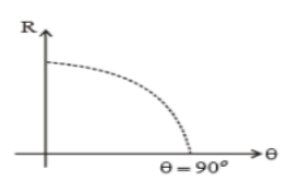2.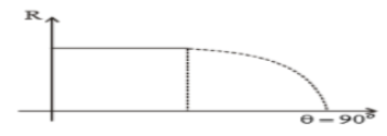3.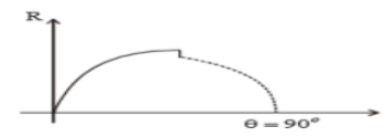(4) None of these

Concept Questions :-

Application of laws
High Yielding Test Series + Question Bank - NEET 2020

Difficulty Level:

A block is placed on a rough horizontal plane. A time dependent horizontal force F=kt acts on the block. The acceleration time graph of the block is :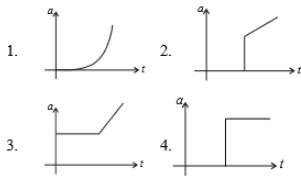Concept Questions :-

Application of laws
High Yielding Test Series + Question Bank - NEET 2020

Difficulty Level:

A particle is moving in a vertical circle. The tension in the string when passing through two positions at anele of 30$°$ and 60$°$ from vertical (the lowest position) are respectively, then:

1.

2.

3.

4.  Tension in the string always remains the same.

Concept Questions :-

Non-Uniform vertical circular motion
High Yielding Test Series + Question Bank - NEET 2020

Difficulty Level:

A motorcycle is going on an overbridge of radius R. The driver maintains a constant speed. As the motorcycle is ascending on the overbridge, the normal force on it:

1.  Increases

2.  decreases

3.  remains the same

4.  fluctuates erratically

Concept Questions :-

Non-Uniform vertical circular motion
High Yielding Test Series + Question Bank - NEET 2020

Difficulty Level:

A rod of length L and mass m is acted on by two unequal forces ${F}_{1}$ and ${F}_{2}\left(<{F}_{1}\right)$ as shown in the following figure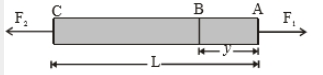The tension in the rod at a distance y from the end A is given by :

1. ${F}_{1}\left(1-\frac{y}{L}\right)+{F}_{2}\left(\frac{y}{L}\right)$

2. ${F}_{2}\left(1-\frac{y}{L}\right)+{F}_{1}\left(\frac{y}{L}\right)$

3. $\left({F}_{1}-{F}_{2}\right)\frac{y}{L}$

4. None of the above

Concept Questions :-

Application of laws
High Yielding Test Series + Question Bank - NEET 2020

Difficulty Level:

Two blocks A and B of masses m & 2m respectively are held at rest such that the spring is in natural length. Find the accelerations of both the blocks just after release.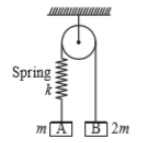(1)

(2)

(3) (0, 0)

(4)

Concept Questions :-

Application of laws
High Yielding Test Series + Question Bank - NEET 2020

Difficulty Level:

Two blocks 'A' and 'B' each of mass 'm' are placed on a smooth horizontal surface. Two horizontal force F and 2F are applied on both the blocks 'A' and 'B' respectively as shown in figure. The block A does not slide on block B. Then the normal reaction acting between the two blocks is: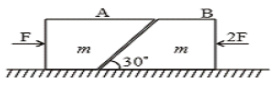(1) F

(2) F/2

(3) $\frac{F}{\sqrt{3}}$

(4) 3F

Concept Questions :-

Application of laws